# Consider the following reactions:

Question:

Consider the following reactions: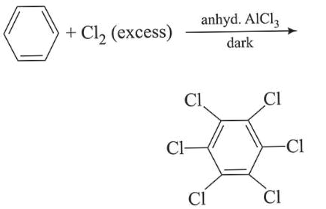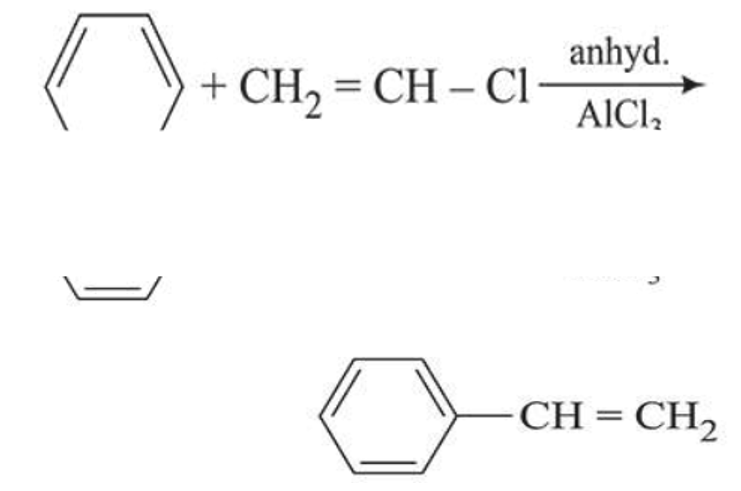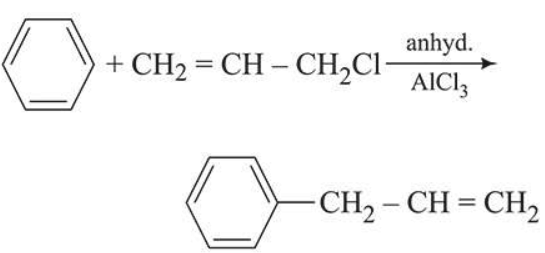Which of these reactions are possible?

1. (A) and (B)

2. (A) and (D)

3.

(B), (C) and (D)

4. (B) and (D)

Correct Option:

Solution:

The plots of radial distribution functions for various orbitals of hydrogen atom against 'r' are given below: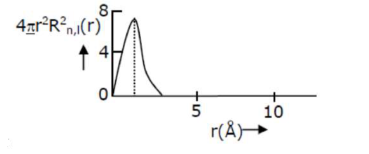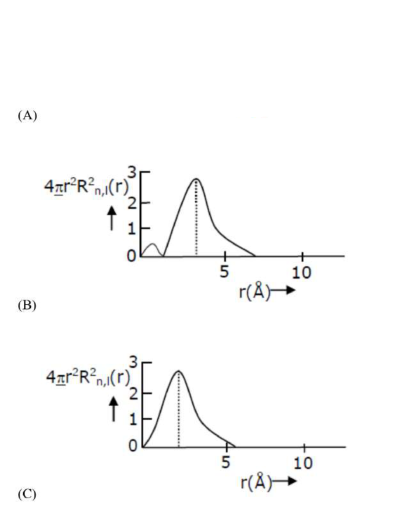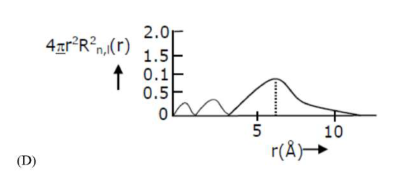The correct plot for $3 \mathrm{~s}$ orbital is:

(1) $\mathrm{D}$

(2) B

(3) $\mathrm{A}$

(4) $\mathrm{C}$